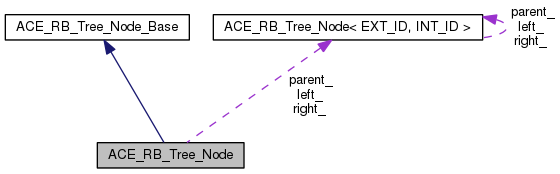ACE  6.0.6
ACE_RB_Tree_Node Class Reference

Implements a node in a Red-Black Tree ADT. More...

`#include <RB_Tree.h>`

Inheritance diagram for ACE_RB_Tree_Node:[legend]
Collaboration diagram for ACE_RB_Tree_Node:[legend]

List of all members.

## Public Member Functions

ACE_RB_Tree_Node (const EXT_ID &k, const INT_ID &t)
Constructor.
~ACE_RB_Tree_Node (void)
Destructor.
EXT_ID & key (void)
Key accessor.
INT_ID & item (void)
Item accessor.
void color (RB_Tree_Node_Color c)
Set color of the node.
RB_Tree_Node_Color color (void)
Get color of the node.
ACE_RB_Tree_Node< EXT_ID,
INT_ID > *
parent (void)
Accessor for node's parent pointer.
void parent (ACE_RB_Tree_Node< EXT_ID, INT_ID > *p)
Mutator for node's parent pointer.
ACE_RB_Tree_Node< EXT_ID,
INT_ID > *
left (void)
Accessor for node's left child pointer.
void left (ACE_RB_Tree_Node< EXT_ID, INT_ID > *l)
Mutator for node's left child pointer.
ACE_RB_Tree_Node< EXT_ID,
INT_ID > *
right (void)
Accessor for node's right child pointer.
void right (ACE_RB_Tree_Node< EXT_ID, INT_ID > *r)
Mutator for node's right child pointer.

## Private Attributes

EXT_ID k_
The key.
INT_ID t_
The item.
RB_Tree_Node_Color color_
Color of the node.
ACE_RB_Tree_Node< EXT_ID,
INT_ID > *
parent_
Pointer to node's parent.
ACE_RB_Tree_Node< EXT_ID,
INT_ID > *
left_
Pointer to node's left child.
ACE_RB_Tree_Node< EXT_ID,
INT_ID > *
right_
Pointer to node's right child.

## Detailed Description

Implements a node in a Red-Black Tree ADT.

## Constructor & Destructor Documentation

 ACE_RB_Tree_Node::ACE_RB_Tree_Node ( const EXT_ID & k, const INT_ID & t )

Constructor.

 ACE_RB_Tree_Node::~ACE_RB_Tree_Node ( void )

Destructor.

## Member Function Documentation

 void ACE_RB_Tree_Node::color ( RB_Tree_Node_Color c )

Set color of the node.

 RB_Tree_Node_Color ACE_RB_Tree_Node::color ( void )

Get color of the node.

 INT_ID& ACE_RB_Tree_Node::item ( void )

Item accessor.

 EXT_ID& ACE_RB_Tree_Node::key ( void )

Key accessor.

 ACE_RB_Tree_Node* ACE_RB_Tree_Node::left ( void )

Accessor for node's left child pointer.

 void ACE_RB_Tree_Node::left ( ACE_RB_Tree_Node< EXT_ID, INT_ID > * l )

Mutator for node's left child pointer.

 ACE_RB_Tree_Node* ACE_RB_Tree_Node::parent ( void )

Accessor for node's parent pointer.

 void ACE_RB_Tree_Node::parent ( ACE_RB_Tree_Node< EXT_ID, INT_ID > * p )

Mutator for node's parent pointer.

 ACE_RB_Tree_Node* ACE_RB_Tree_Node::right ( void )

Accessor for node's right child pointer.

 void ACE_RB_Tree_Node::right ( ACE_RB_Tree_Node< EXT_ID, INT_ID > * r )

Mutator for node's right child pointer.

## Member Data Documentation

 RB_Tree_Node_Color ACE_RB_Tree_Node::color_` [private]`

Color of the node.

 EXT_ID ACE_RB_Tree_Node::k_` [private]`

The key.

 ACE_RB_Tree_Node* ACE_RB_Tree_Node::left_` [private]`

Pointer to node's left child.

 ACE_RB_Tree_Node* ACE_RB_Tree_Node::parent_` [private]`

Pointer to node's parent.

 ACE_RB_Tree_Node* ACE_RB_Tree_Node::right_` [private]`

Pointer to node's right child.

 INT_ID ACE_RB_Tree_Node::t_` [private]`

The item.

The documentation for this class was generated from the following file: